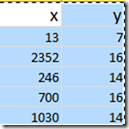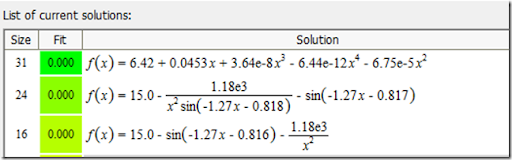## Saturday, August 13, 2011

### Applying artificial intelligence to a brain teaser

Here goes the 14th Brain Teaser. It is a tough maths question and i'm not even sure if the answer is correct. Hopefully i don't lose the site name. Here goes:

If you use a certain formula on 13, you end up with 7.
Under the same formula, 2352 becomes 16, 246 becomes 14, 700 turns into 16, and 1030 becomes 14.
What would 9304 become?

So I decided to test some the Eureqa software, which is some software from Cornell University that test out a bunch of equations to see which ones fit.  Eureqa software is available free from http://creativemachines.cornell.edu/eureqa_download

We enter the data aboveEureqa rapidly finds several functions that fit with zero error.We can then use one of these equations to determine what 9304 would become. Let’s pick the first one, since for sure there is no use of trig functions in the average brain teaser! If this equation were true, 9304 would be –24345.With the thousands of things Eureqa tries, and with only 5 data points, its not surprising that there are several functions which fit the data -- none of which are likely to be the answer famer intended!

#### 1 comment:

1.A commenter on chess.com notes the trick here:

"The first is simply the Lagrange polynomial for those 5 points, which by definition is a *perfect match*. But there are hundreds of functions that pass through those 5 points, e.g. to that 4th degree polynomial you can add *any* >=5th degree polynomial that has those five x-values as roots, and it would still be a solution. You can do the same with trig functions instead of simple polynomials, as your software did, no surprise there.

"And the values you give look very similar to the old count-the-letters trick, but not exactly, i.e. 1030 = thousandthirty = 14 letters, 700 = sevenhundred = 12 letters, 13 = thirteen = 8 letters."